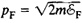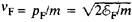# Fermi Level

Also found in: Dictionary.

## Fermi level

[′fer·mē ‚lev·əl]
(statistical mechanics)
The energy level at which the Fermi-Dirac distribution function of an assembly of fermions is equal to one-half. Also known as Fermi energy.
McGraw-Hill Dictionary of Scientific & Technical Terms, 6E, Copyright © 2003 by The McGraw-Hill Companies, Inc.
The following article is from The Great Soviet Encyclopedia (1979). It might be outdated or ideologically biased.

## Fermi Level

the energy level below which all energy states of the particles of a degenerate gas that obey Fermi-Dirac statistics are occupied at a temperature of absolute zero (seeSTATISTICAL MECHANICS). Particles of a degenerate gas that obey Fermi-Dirac statistics are called fermions. The existence of the Fermi level is a consequence of the Pauli exclusion principle, according to which not more than (2s + 1) particles, where s is the spin of a particle, may occupy a state with a specified momentum p. The Fermi level coincides with the values of the chemical potential of a fermion gas at T = 0°K.

The Fermi level ℰF can be expressed in terms of the number n of gas particles per unit volume: ℰF = [(2πℏ)2/2m] [3n/4π(2s + 1)], where m is the particle mass. The quantityis called the Fermi momentum. At T = 0°K, the particles occupy all states with momenta p < pF; all states with p > pF are empty. In other words, at T = 0°K, fermions occupy states in momentum space that lie within a sphere p2 = 2mF of radius pF; this sphere is called the Fermi sphere. Upon heating, some particles move from a state with p < pF to a state with p > pF. Vacant sites, called holes, occur within the Fermi sphere. The quantityis called the Fermi velocity and specifies the upper limit to the velocities of fermions at T = 0°K.

A degenerate gas consisting of conduction electrons in a solid at T = 0°K occupies surfaces of more complex shape in momentum space (see).

### REFERENCE

Landau, L. D., and E. M. Lifshits. Statisticheskaia fizika, 2nd ed. Moscow, 1964. (Teoreticheskaia fizika, vol. 5.)

M. I. KAGANOV

References in periodicals archive ?
Our results show that the Fermi level is displaced towards the CB, and for the bulk and surface, the GGA method delocalizes the electron in the conduction band.
Multilayer's Fermi level shift by Kelvin probe force microscopy (KPFM) technique is also determinate and the obtained results were correlated with optical transmission spectra measurements.
The conduction band is narrowed, which indicates that the Sn[O.sub.2] model after different ratios of doping enhances the electronic state near the Fermi level, and more partial waves cross the Fermi level, the interaction between electrons is enhanced, and the conductivity is enhanced.
Near the Fermi level, the variation of DOS at the bottom of the conduction band is relatively smooth.
As the change of DOS For [C.sub.6][H.sub.6] sensing shown in Figures 3(a) and 3(b), the TDOS near the Fermi level tends to be volatile especially below and above the Fermi level, where it is obviously decreased.
The transmission coefficients at the Fermi level for spin-up and spin-down electrons are 0.41 and 0.09 G0, respectively.
is the Fermi level of the matrix in the intrinsic region; [E.sub.c] and [E.sub.[upsilon]] are the energy levels of the conduction band and the valence band, respectively, of the matrix; [N.sub.c] and [N.sub.[upsilon]] are the number density of states of the electrons and the holes, respectively.
The obtained results give the information on the band gap, its nature, and probability of finding the electron near the Fermi level. The calculated band gaps of all the GaN nanotubes taken into consideration are reported in Table 1 and discussed in the following sections.
In this system the local electron density is determined by the Fermi level which is a property of the solid as a whole.
The electronic energy levels of [C.sub.28] and Li@[C.sub.28] molecules near Fermi level are illustrated in Figure 3.

Site: Follow: Share:
Open / Close